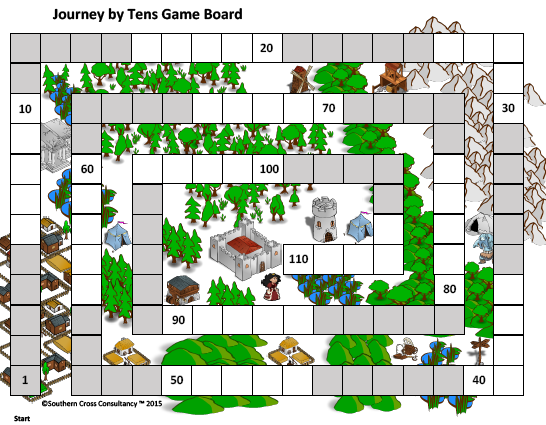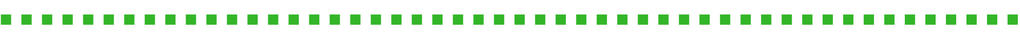Math Resources and Games# Grade 1 Math CCSS Games Packet (over 100+ Games!)

\$14.95

In Grade 1, instructional time should focus on four critical areas:

(1) developing understanding of addition, subtraction, and strategies for addition and subtraction within 20;

(2) developing understanding of whole number relationships and place value, including grouping in tens and ones;

(3) developing understanding of linear measurement and measuring lengths as iterating length units; and

(4) reasoning about attributes of, and composing and decomposing geometric shapes.

Southern Cross Consultancy have developed over 100 hands-on games, Math centers and activities for use in the classroom utilizing the "I Can" format to reinforce the student-friendly instructions, reinforcing student independence.

The Grade 1 Math CCSS Games Packet includes:OPERATIONS AND ALGEBRAIC THINKING
Represent and solve problems involving addition and subtraction.

1OA1. Use addition and subtraction within 20 to solve word problems involving situations of adding to, taking from, putting together, taking apart, and comparing, with unknowns in all positions, e.g., by using objects, drawings, and equations with a symbol for the unknown number to represent the problem.

Add To Change Unknown to 10*
Add To Change Unknown to 20*
Add To Result Unknown to 10
Add To Result Unknown to 20*
Add To Start Unknown to 10
Add To Start Unknown to 20*
Compare Bigger Unknown Fewer Version to 20*
Compare Bigger Unknown More Version to 20*
Compare Difference Unknown How Many Fewer Version to 20*
Compare Difference Unknown How Many More Version to 20*
Compare Smaller Unknown Fewer Version to 20*
Compare Smaller Unknown More Version to 20*
Popsicle Stick Subtraction Game up to 20*
Popsicle Stick Subtraction Game up to 9*
Put Together Take Apart Addend Unknown to 10*
Put Together Take Apart Addend Unknown to 20*
Put Together Take Apart Both Addends Unknown to 20*
Put Together Take Apart Total Unknown to 20*
Story Problem Sentence Frame Change Unknown*
Take From Change Unknown to 10*
Take From Change Unknown to 20*
Take from Result Unknown to 10*
Take From Result Unknown to 20*
Take From Start Unknown to 10*
Take From Start Unknown to 20*

1OA2. Solve word problems that call for addition of three whole numbers whose sum is less than or equal to 20, e.g., by using objects, drawings, and equations with a symbol for the unknown number to represent the problem. One Watermelon Seed (www.amazon.com)
Understand and apply properties of operations and the relationship between addition and subtraction.
1OA3. Apply properties of operations as strategies to add and subtract. Examples: If 8 + 3 = 11 is known, then 3 + 8 = 11 is also known. (Commutative property of addition.) To add 2 + 6 + 4, the second two numbers can be added to make a ten, so 2 + 6 + 4 = 2 + 10 = 12. (Associative property of addition.) Domino Turn Arounds
Turn Around Facts*
1OA4. Understand subtraction as an unknown-addend problem. For example, subtract 10 – 8 by finding the number that makes 10 when added to 8. Addition Subtraction Heads Up*
How Many are Hiding Under the Cup?
How Many Are Hiding?*
Subtraction Bag*
1OA5. Relate counting to addition and subtraction (e.g., by counting on 2 to add 2).

1 More Than, 1 Less Than Bingo to 20
Count on 1 Bingo*
Count on 1, 2, 3, 0 Bingo*
Two More, Two Less*

1OA6. Add and subtract within 20, demonstrating fluency for addition and subtraction within 10. Use strategies such as counting on; making ten (e.g., 8 + 6 = 8 + 2 + 4 = 10 + 4 = 14); decomposing a number leading to a ten (e.g., 13 – 4 = 13 – 3 – 1 = 10 – 1 = 9); using the relationship between addition and subtraction (e.g., knowing that 8 + 4 = 12, one knows 12 – 8 = 4); and creating equivalent but easier or known sums (e.g., adding 6 + 7 by creating the known equivalent 6 + 6 + 1 = 12 + 1 = 13).

5 Plus, Plus 5*
Anything but 8*
Buzz Off*
Closest to 20*
Domino Doubles*
Domino Facts *
Double the Card*
Double the Number*
Doubles Facts Poster*
Easy Up Easy Down*
Five in a Row*
Go Fish to 10
Make 10 Race*
Make 10 with a Ten Frame*
Make 10*
Make 20 with Ten Frames*
Pairs of Ten*
Plus 1 or Plus 2 Bingo*
Roll to Zero from 10*
Roll to Zero from 20*
Score the Difference*
Subtract 1 or 2*
Subtract Up or Down*
Take From Ten*
Ten Frame Fact Families*
The 9 vs 10 Race*
The Number Target Game*
Tiered Count on 1 Games*
Tiered Count on 2 Games*
Tiered Count on 3 Games*
Tiered Doubles +1 Games*
Tiered Doubles -1 Games*
Tiered Doubles Games*
Top It Dominoes*
Walk the Dog Domino Game*
What Else Makes 10?*
What's the Difference?*

Work with addition and subtraction equations.
1OA7. Understand the meaning of the equal sign, and determine if equations involving addition and subtraction are true or false. For example, which of the following equations are true and which are false? 6 = 6, 7 = 8 – 1, 5 + 2 = 2 + 5, 4 + 1 = 5 + 2. Sorting True or False*
True or Not True
1OA8. Determine the unknown whole number in an addition or subtraction equation relating three whole numbers. For example, determine the unknown number that makes the equation true in each of the equations 8 + ? = 11, 5 = _ – 3, 6 + 6 = _. Complete the Equation
NUMBER AND OPERATIONS IN BASE TEN
Extend the counting sequence.
1NBT1. Count to 120, starting at any number less than 120. In this range, read and write numerals and represent a number of objects with a written numeral. Count Down
Counting on the Grid*
Forwards Counting From Any Decades Number 19-89*
Forwards Counting From Any Number 16-69*

Understand place value.
1NBT2. Understand that the two digits of a two-digit number represent amounts of tens and ones. Understand the following as special cases:

1NBT2a. 10 can be thought of as a bundle of ten ones — called a “ten.”

1NBT2b. The numbers from 11 to 19 are composed of a ten and one, two, three, four, five, six, seven, eight, or nine ones.

1NBT2c. The numbers 10, 20, 30, 40, 50, 60, 70, 80, 90 refer to one, two, three, four, five, six, seven, eight, or nine tens (and 0 ones).

Groups of Ten*
Place Value Dominoes*
Trading Game Tens and Ones Base 10 Blocks
Trading Game Tens Ones Bundling Sticks and Elastic Bands
Two Digits Expanded Match*

1NBT3. Compare two two-digit numbers based on meanings of the tens and ones digits, recording the results of comparisons with the symbols >, =, and <. Flash*
Vertical Number Line
Use place value understanding and properties of operations to add and subtract.
1NBT4. Add within 100, including adding a two-digit number and a one-digit number, and adding a two-digit number and a multiple of 10, using concrete models or drawings and strategies based on place value, properties of operations, and/or the relationship between addition and subtraction; relate the strategy to a written method and explain the reasoning used. Understand that in adding two-digit numbers, one adds tens and tens, ones and ones; and sometimes it is necessary to compose a ten. Add 10 More*
First to 100 Wins
Mystery Number Game*
Plus 10*
1NBT5. Given a two-digit number, mentally find 10 more or 10 less than the number, without having to count; explain the reasoning used. 10 More, 10 Less
Journey by 10's*
Subtract 10*
1NBT6. Subtract multiples of 10 in the range 10-90 from multiples of 10 in the range 10-90 (positive or zero differences), using concrete models or drawings and strategies based on place value, properties of operations, and/or the relationship between addition and subtraction; relate the strategy to a written method and explain the reasoning used. Subtract 10 from Multiples of 10
MEASUREMENT AND DATA
Measure lengths indirectly and by iterating length units.
1MD1. Order three objects by length; compare the lengths of two objects indirectly by using a third object. Comparing and Ordering Lengths
1MD2. Express the length of an object as a whole number of length units, by laying multiple copies of a shorter object (the length unit) end to end; understand that the length measurement of an object is the number of same-size length units that span it with no gaps or overlaps. Limit to contexts where the object being measured is spanned by a whole number of length units with no gaps or overlaps. Estimate and Measure*
Longer or Shorter Names
Tell and write time and money.

1MD3. Tell and write time in hours and half-hours using analog and digital clocks.

Recognise and identify coins, their names, and their value.

Penny Dime Dollar Exchange*
Penny Nickel Dime Exchange*
Penny Nickel Dime Quarter Exchange*
Penny Nickel Exchange
Time Bump Set A*
Time Bump Set B*
Time is of the Essence*
Represent and interpret data.
1MD4. Organize, represent, and interpret data with up to three categories; ask and answer questions about the total number of data points, how many in each category, and how many more or less are in one category than in another.
GEOMETRY
Reason with shapes and their attributes
1G1. Distinguish between defining attributes (e.g., triangles are closed and three-sided) versus non-defining attributes (e.g., color, orientation, overall size); build and draw shapes to possess defining attributes. Shape Sort
Squares and Not Squares*
Toothpick Shapes*
Triangles and Not Triangles*
What’s My Shape*
1G2. Compose two-dimensional shapes (rectangles, squares, trapezoids, triangles, half-circles, and quarter-circles) or three-dimensional shapes (cubes, right rectangular prisms, right circular cones, and right circular cylinders) to create a composite shape, and compose new shapes from the composite shape. Different Shapes with 2 Triangles*
Different Sized Squares*
Different Ways to Cover a Hexagon*
Geo-board Shapes
Mosaic Puzzle Version 1*
Mosaic Puzzle Version 2*
Mosaic Puzzle Version 3*
Mosaic Puzzle Version 4*
Pattern Block Puzzles
1G3. Partition circles and rectangles into two and four equal shares, describe the shares using the words halves, fourths, and quarters, and use the phrases half of,fourth of, and quarter of. Describe the whole as two of, or four of the shares. Understand for these examples that decomposing into more equal shares creates smaller shares.